# 面向对象第一单元博客作业

### 面向对象第一单元总结博客

#### PART ONE 架构相关（UML类图与耦合度分析）

UML类图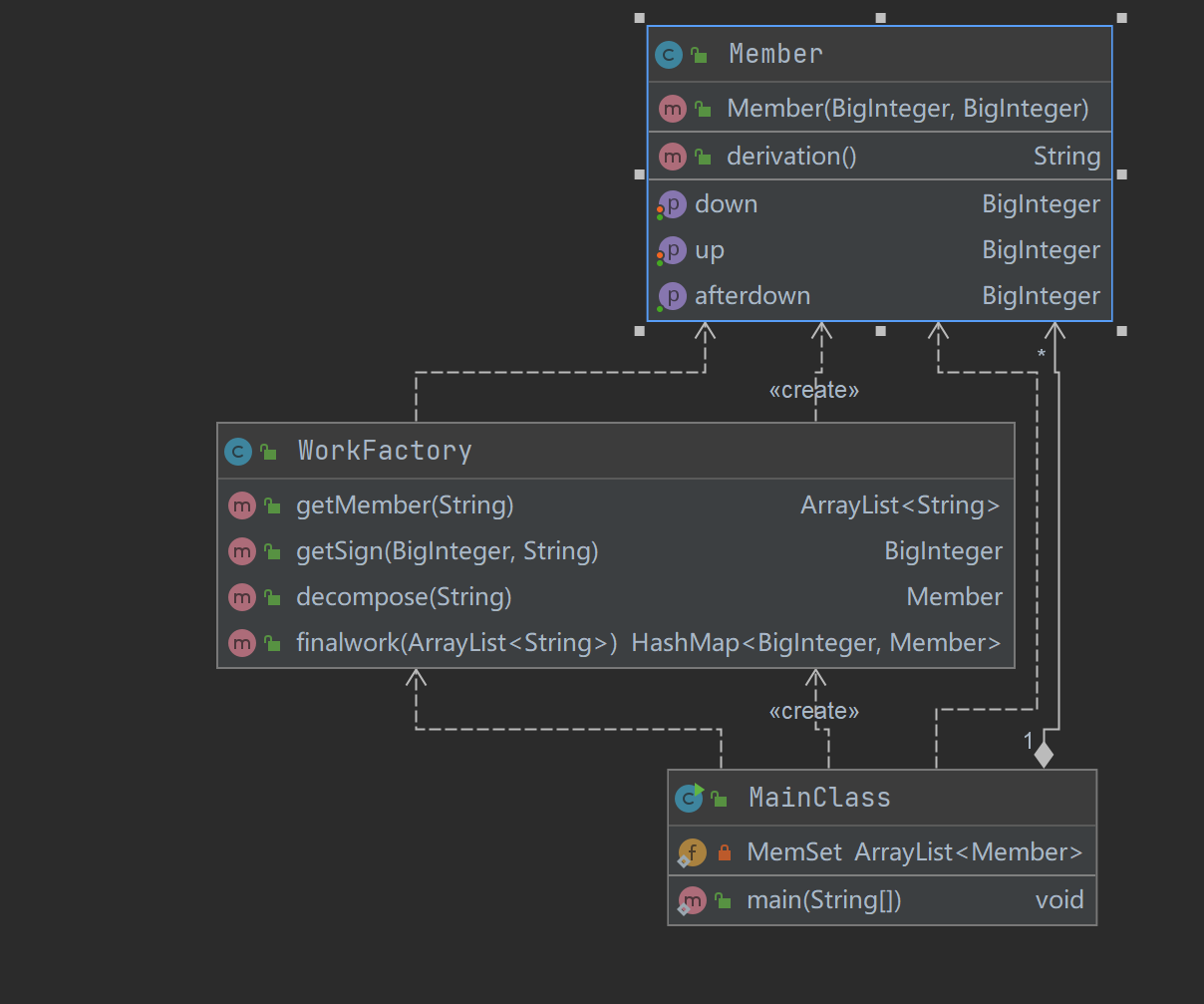Method metrics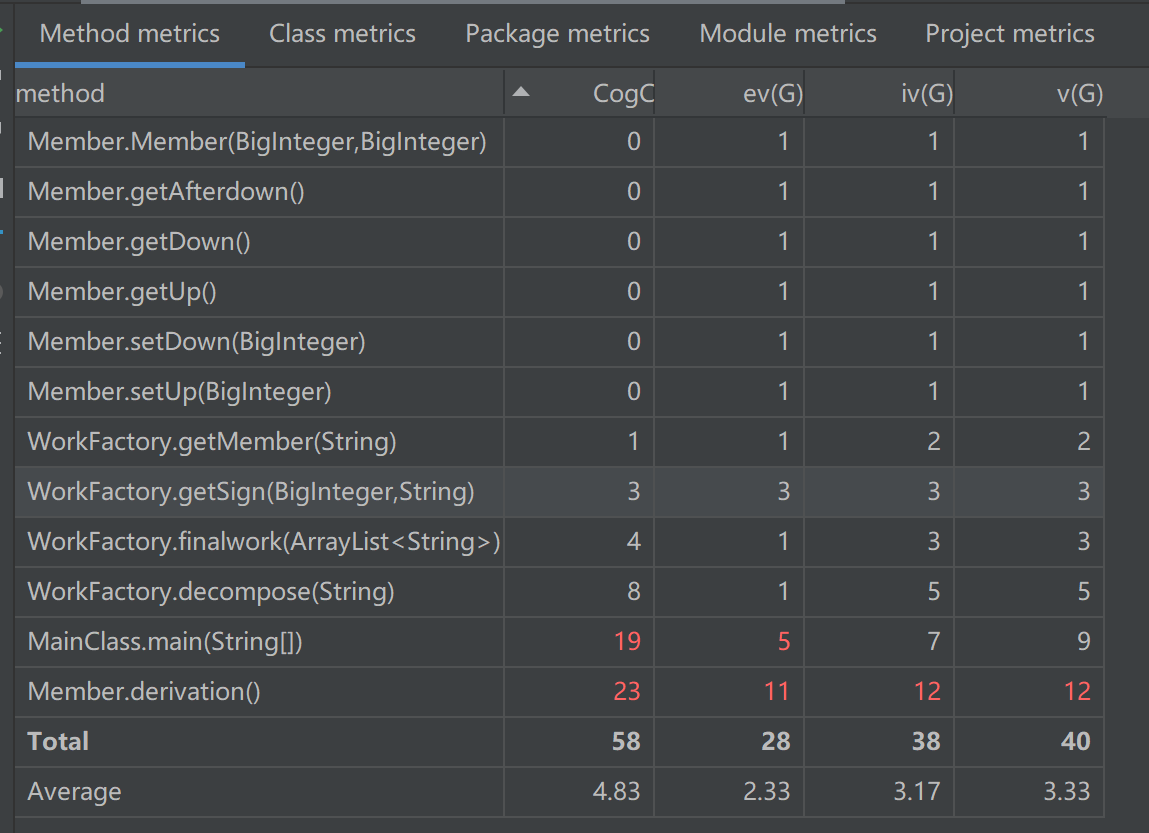Class metrics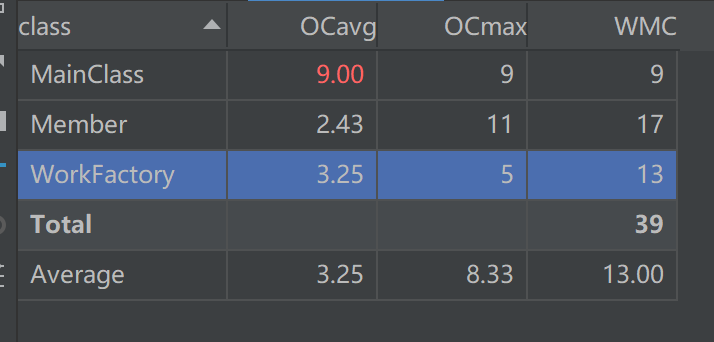UML类图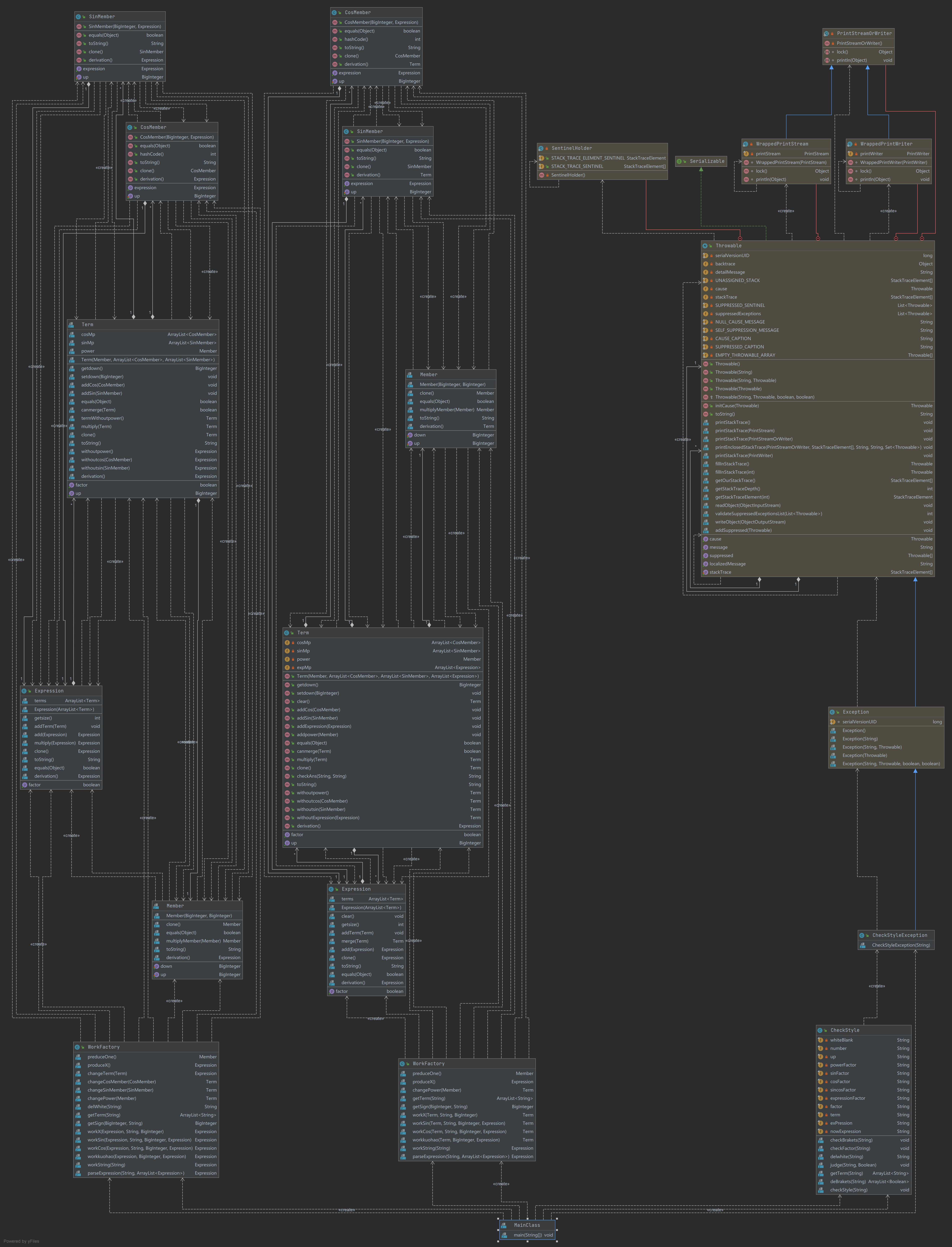Class Metrics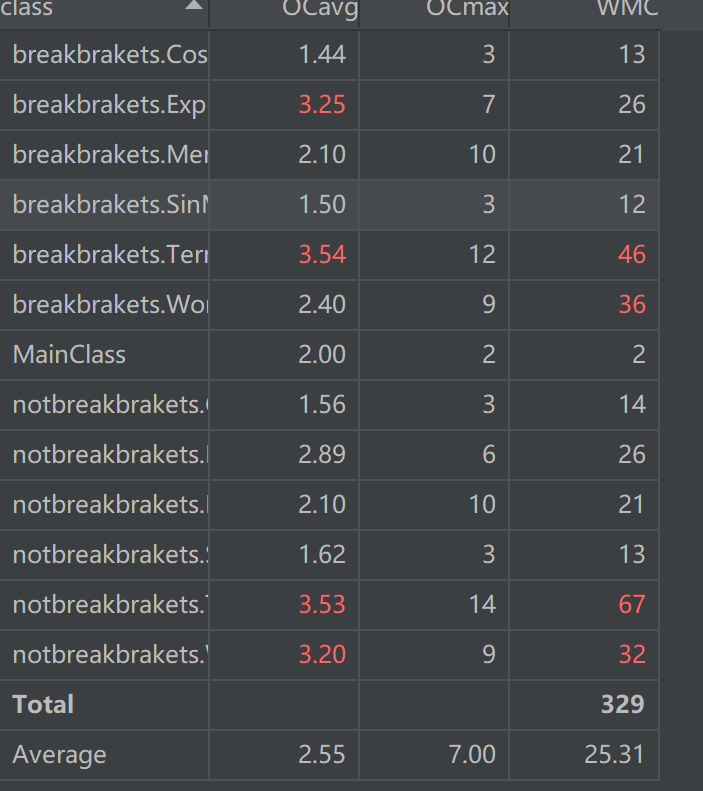UML类图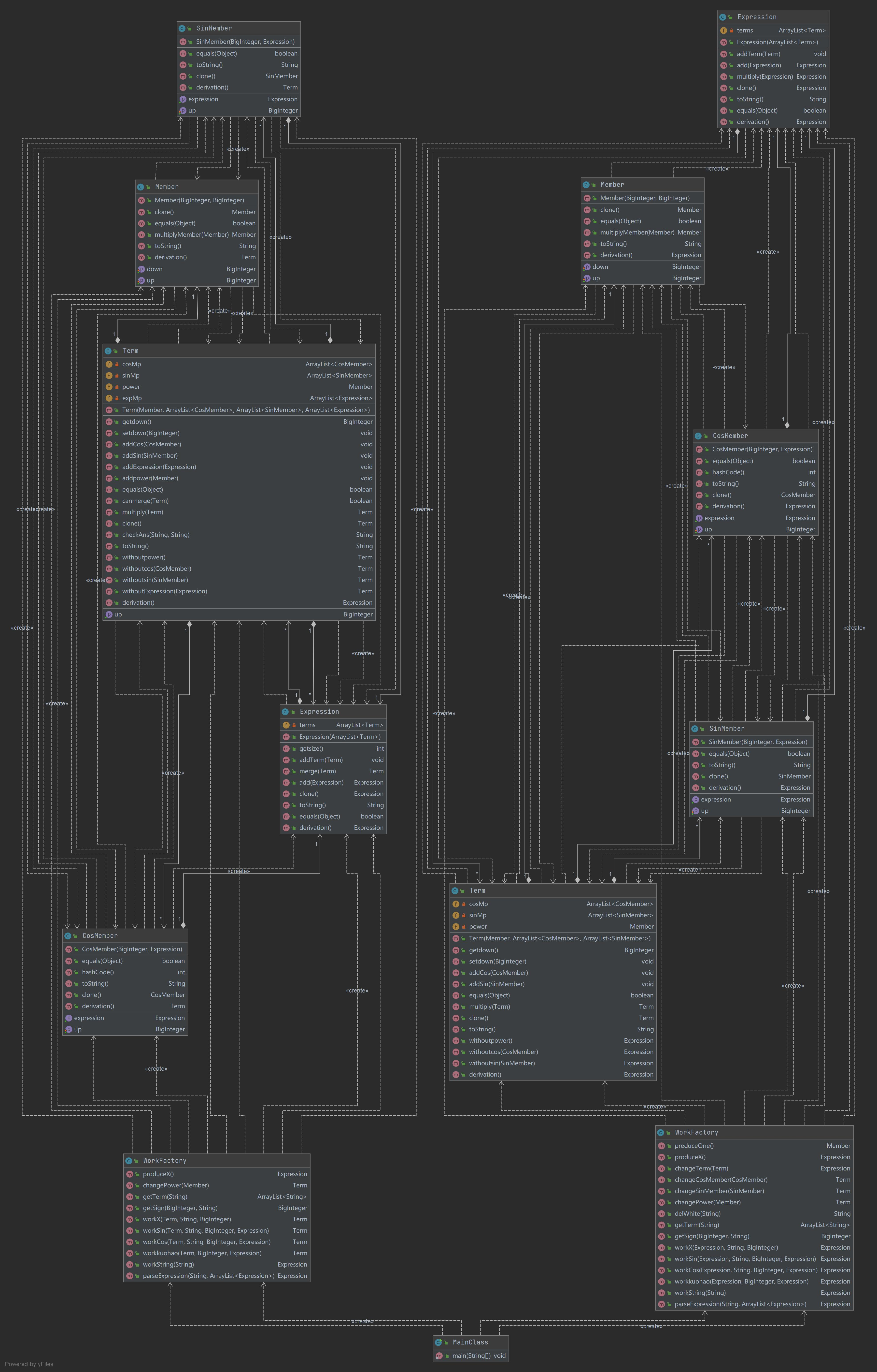Class Metrics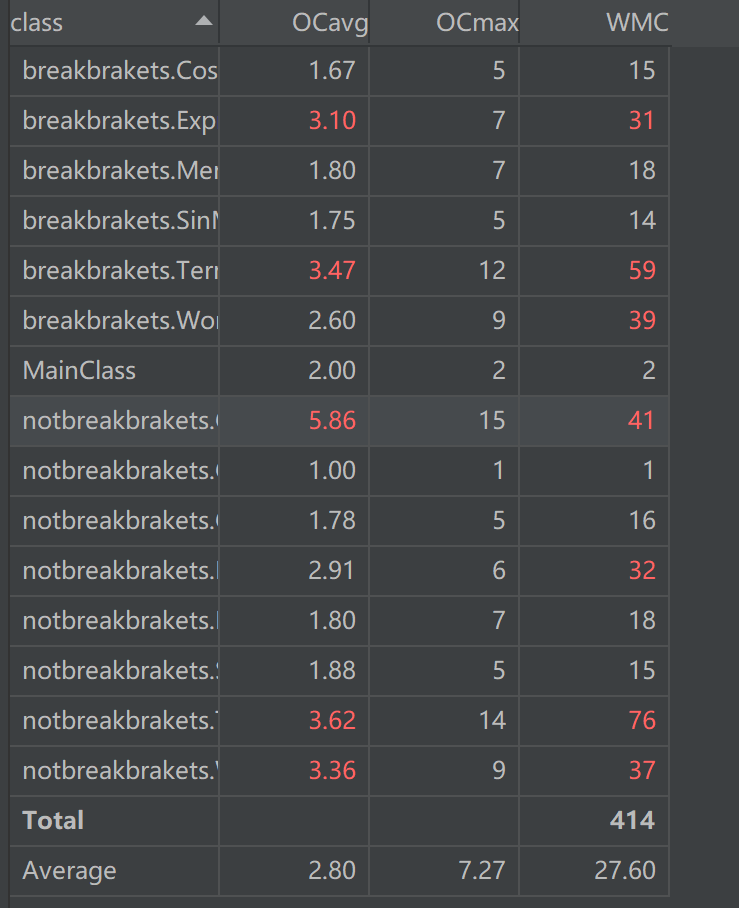emmm……，真惨（，循环复杂度高，大概是因为我用的所有的容器都是 Arraylist 吧，基本所有操作都是循环完成的，复杂度轻轻松松冲上 $$O(n^2)$$ ，此外由于并没有用层次结构，存在一些暴力枚举和暴力判断。其实本来还觉得自己写的不错，但是看了这个分析，我发现我写的万一原来是垃圾，希望以后的作业中能尽量写出一些漂亮的架构。

#### PART TWO 设计与算法相关

(?<member>[+-]?([+-]?([+-]?((\\d+)|(x(\\*\\*[+-]?\\d+)?))\\*)*[+-]?((\\d+)|(x(\\*\\*[+-]?\\d+)?))))


$\sum kx^p*f_1(x)*f_2(x)*f_3(x)…*f_m(x)*(…)_1*(…)_2…(…)_n \\ k,p,m,n \in N$

$\sum kx^p*f_1()*f_2()*f_3()…*f_m()*()_1*()_2…()_n \\ k,p,m,n \in N$

$(\prod f_1(x)*f_2(x)…f_n(x))^{'} =(f_1(x))^{'}*f_2(x)…f_n(x)+(f_2(x))^{'}*f_1(x)…f_n(x)+(f_n(x))^{'}*f_1(x)…f_{n-1}(x)$

$sin(f(x))^{'} = cos(f(x))*f(x)^{'} \\ cos(f(x))^{'} = -sin(f(x))*f(x)^{'}$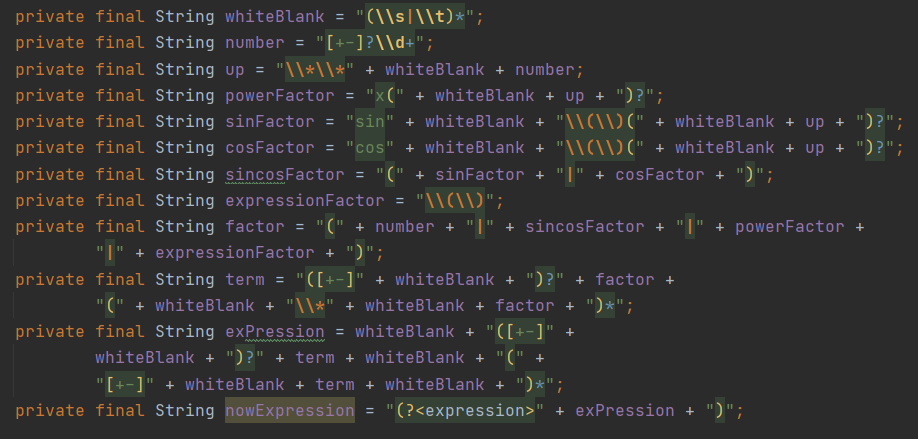• 先对所有括号进行匹配，如果括号无法匹配，直接抛出异常
• 去除表达式前后的空格，之后判断表达式是否为空，如果为空，直接抛出异常。
• 做括号匹配，同之前的一样，提取括号中的表达式，之后对处理后的串做匹配。这里需要注意一下，这里表达式需要分为三角函数中的表达式和不同的表达式，可以用一个参数 id 来表示，如果是三角函数中的表达式，需要特殊判断它是否是一个因子。
• 如果表达式合法，解析表达式，判断当前表达式中每一对括号的归属，也就是计算 id，然后对从括号中提取的表达式进行递归判断。

#### PART THREE 心得和体会

• 取消愚蠢的 “双版本” 模式，将两种版本融合，实际上，可以直接在 “项” 类和 "表达式" 类中开一种方法表示拆括号的情况，解析表达式，求导等步骤之前都采用不拆括号的模式处理，之后再判断得到的结果查括号更短还是不拆括号更短。
• 开一个抽象类表示因子维护，指数 ，获取指数，展示指数，clone，toString ，derivative 等方法，其它四类因子继承这个抽象类，这样就方便 ”项“ 类统一管理，相较于我现在的版本可以减少很多码量。

OO第一次作业结束了，太tm过瘾了，期待可以在以后的OO学习中加深对面向对象方法的理解，提升我的写代码能力，成为一个更加成熟的程序猿。

posted @ 2021-03-26 17:53  iuiou  阅读(144)  评论(4编辑  收藏  举报